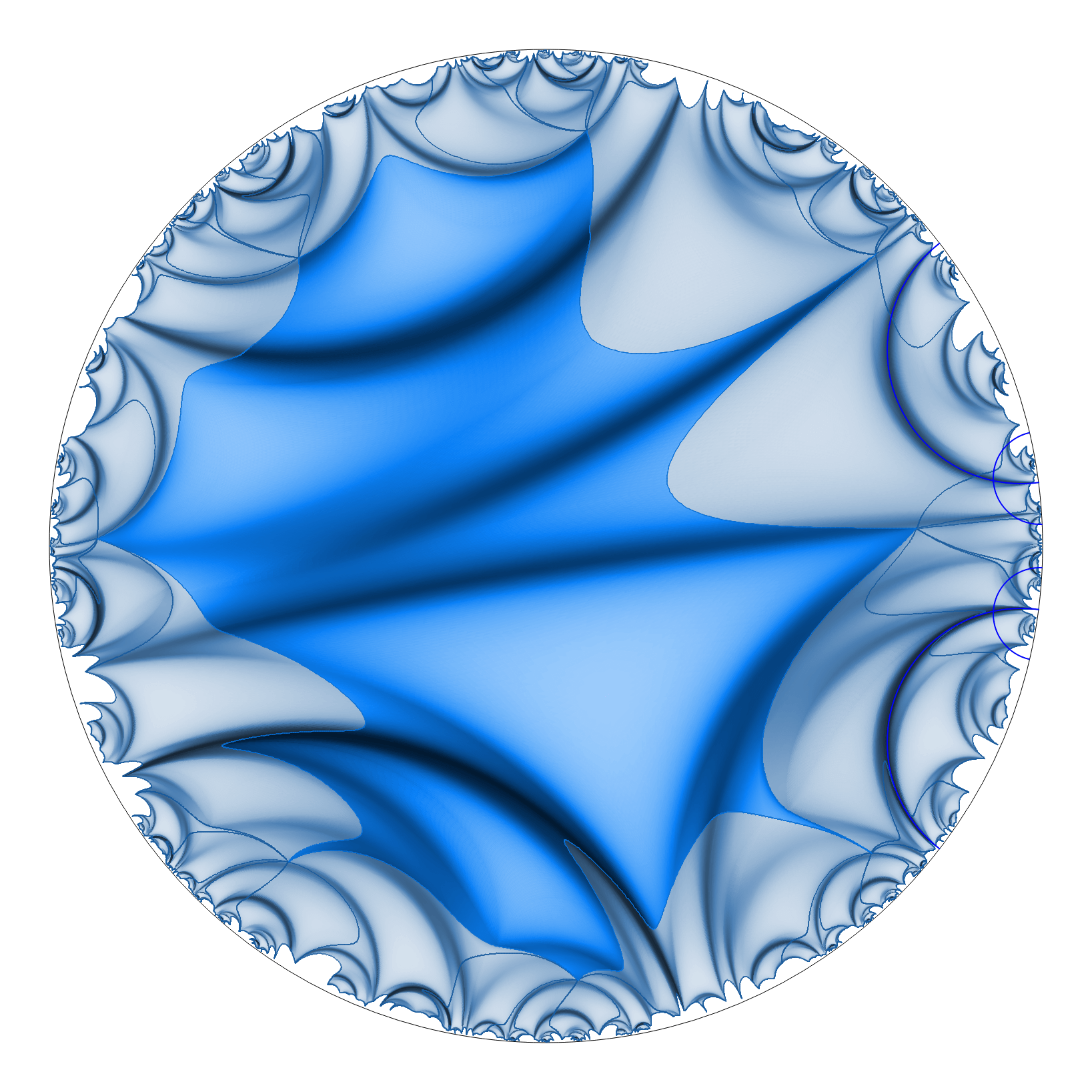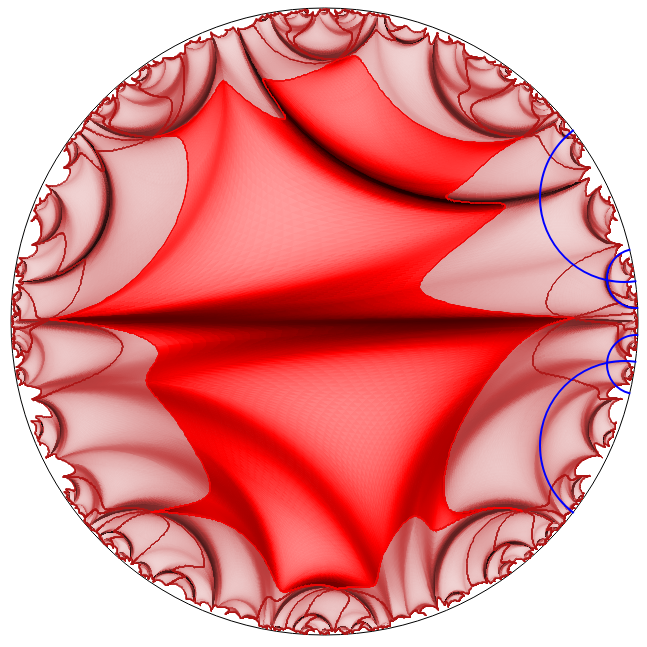Research interests

Teichmüller-Thurston theory, hyperbolic geometry, Riemannian geometry, symplectic geometry of moduli spaces, complex analysis and geometry, minimal surfaces and harmonic maps, geometric analysis, discrete differential geometry, mathematical programming, mathematics visualization, mathematics education.

Publications

Published articles and preprints

1. The complex symplectic geometry of the deformation space of complex projective structures.
2. Minimal surfaces and symplectic structures of moduli spaces.
3. Bi-Lagrangian structures and Teichmüller theory (with Andy Sanders).
4. Computing discrete equivariant harmonic maps (with Jonah Gaster and Léonard Monsaingeon).
Submitted. Preprint:
5. Computing harmonic maps between Riemannian manifolds (with Jonah Gaster and Léonard Monsaingeon).
Submitted. Preprint:
6. The sum of Lagrange numbers (with Jonah Gaster).
7. Harmonic maps from Kähler manifolds.
1. Hyper-Kähler geometry of the Taubes moduli space (with Francesco Bonsante, Andy Sanders, and Andrea Seppi).
We introduce a mixed signature hyper-Kähler metric on the Taubes moduli space, extending the hyper-Kähler metric of Donaldson off almost-Fuchsian space.
2. Complex geometry of the universal moduli space of Higgs bundles (with Andy Sanders and Nicolas Tholozan).
We study the complex, Kähler and hyper-Kähler geometry of the universal moduli space of Higgs bundles over Teichmüller space.
3. Symplectic geometry of Wick rotations (with Carlos Scarinci).
We study the symplectic properties of Wick rotations between moduli spaces of Einstein 3-manifolds in relation to bi-Lagrangian structures.
4. Discrete Bochner formula on Riemannian manifolds (with {Jonah Gaster} and Léonard Monsaingeon).
We establish a discrete Bochner formula for functions on a weighted triangulation taking values in Riemannian manifold.

Notes

1. Higgs bundles and Hitchin components.
Handwritten written for the workshop Higher Teichmüller-Thurston spaces in Paris XI, France in 2012. Download.
2. Minimal surfaces and quasi-Fuchsian structures.
Notes for the NSF workshop Higgs bundles and harmonic maps in Asheville, NC in Januray 2015. Download.
3. Riemann surfaces.
Lecture notes for a Masters course taught at TU Darmstadt, Winter 2018-2019.
4. Hyperbolic geometry and applications.
Report of the GRG group (HITS). To appear in HITS Annual Report 2020.Book

1. Hyperbolic geometry
This book is based on a Master course taught at TU Darmstadt in the winter 2019-2020. It builds on lecture notes written for a similar course taught at Rutgers University in 2017. Preprint: arXiv:2003.11180 or HAL-02518149. Download.

Ph.D. thesis

I did my doctoral studies at Université de Toulouse III from 2008 to 2011 under the supervision of Jean-Marc Schlenker. Ph.D. thesis: The symplectic geometry of the deformation space of complex projective structures on a surface.

"Ad" : I invite you to consider submitting your most beautiful mathematics papers to the free and open journal Annales Henri Lebesgue. (I have no ties to this journal!)

Computational mathematics

I have had a growing interest for discrete differential geometry, computational mathematics, experimental research, and visualization:

Heidelberg Experimental Geometry Lab (HEGL)

At Heidelberg University and HITS, I have helped set up the Heidelberg Experimental Geometry Lab (HEGL), under the lead of Anna Wienhard. The goal of HEGL is to promote the interaction between theoretical math research and computational experimentation and visualization. HEGL provides advanced technology and hosts many undergraduate research projects.

Go to HEGL website.Circle Packings

This cross-platform computer software created by Benjamin Beeker and myself computes and shows circle packings and Riemann conformal mappings.

Read more in the "Software" section here.

HarmonyThis is an ongoing project with Jonah Gaster.

This cross-platform computer software computes and shows equivariant harmonic maps from the hyperbolic plane $$\mathbb{H}^2$$ to hyperbolic 3-space $$\mathbb{H}^3$$ (or in the future, more general symmetric spaces).

Read more in the "Software" section here.

Supervising student research

1. I supervised the Master thesis of Darja Zierau in the Summer 2019, entitled Cross-ratios of torsion points on elliptic curves.
2. I will supervise several undergraduate research projects at the Heidelberg Experimental Geometry Lab (HEGL) in the Spring 2021: see HEGL website.

CV